# How-to Quickly Mock-up Data for a Dashboard Template

I frequently need to create and test a chart design concept for Excel Dashboards.  Therefore, I need chart data that is representative of the actual data, but I may not have actual data.  And it is also good to have lots of Random data points that will test my concept that may not be in the actual data set but may occur at some point in time.  So how do I do this?

In order to create quick data set for my Excel Charts, I use the Excel function RandBetween()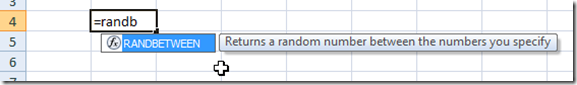As you can see in this picture from an Excel Worksheet, the Function works like this:

It returns a random number between two numbers that you input.

But the both have to be integers and non-negative

=RandBetween(Low End, High End)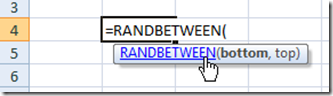Also, remember, that the RandBetween Excel Function returns a NEW random number every time the worksheet is calculated.

So if I need to create a quick chart and test out a Company Dashboard Concept, Tip or Trick, I will type in the RandBetween() Excel function, copy it down and I have my data set.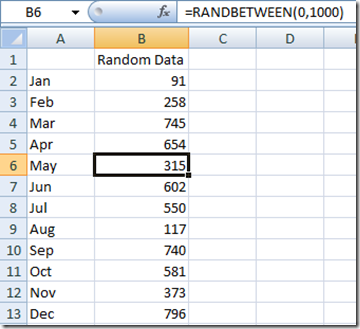Then I can quickly make an Excel chart.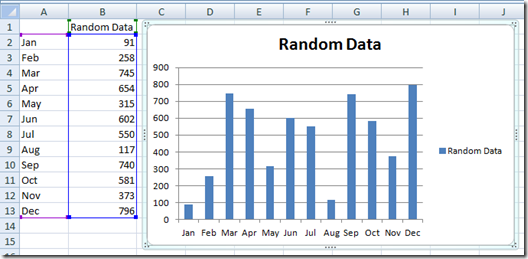Also, I can then use the fact that it changes regularly, I can test see if my chart will have any problems that the first data set didn’t show me.  If I select a cell and click into the formula bar and then press enter, Excel will re-randomize my data and the chart will be updated.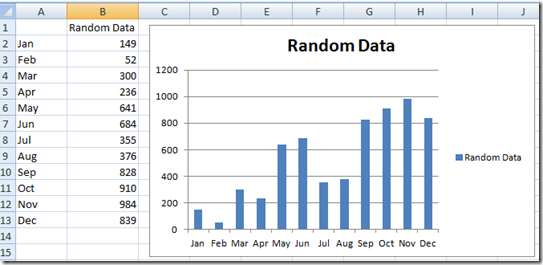Have you used RandBetween Excel Formula before?  Let me know in the comments.

Steve=True